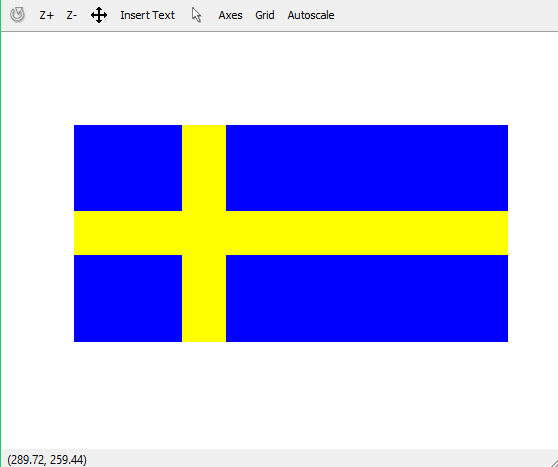Related Articles
Draw Sweden Flag using matlab
• Last Updated : 07 Feb, 2019

A colored image can be represented as a 3 order matrix. The first order is for the rows, the second order is for the columns and the third order is for specifying the color of the corresponding pixel. Here we use the RGB color format, so the third order will take 3 values of Red, Green ans Blue respectively. The values of the rows and columns depends on the size of the image.

Prerequisite : RGB image representation

Approach :

• Make a 3 order zero matrix of dimensions 300X600X3. 300 denotes the number of pixels for rows, 600 denotes number of pixels for columns and 3 denotes the color coding in RGB format.
• Paint the complete image in blue. RGB code for blue is (0, 0, 255)
• Make the horizontal yellow bar. RGB code for yellow is (255, 255, 0)
• Make the vertical yellow bar.

Below is the implementation:

 `% matlab code to draw Sweden flag`` ` `I = zeros(300, 600, 3);`` ` `%painting the whole image blue``I(:, :, 3) = 255; `` ` `%yellow bar``I(120:180, :, 1:2) = 255; I(120:180, :, 3) = 0;`` ` `%yellow column``I(:, 150:210, 1:2) = 255;I(:, 150:210, 3) = 0; `` ` `%print the matrix as image``imshow(I) `

Output:Attention geek! Strengthen your foundations with the Python Programming Foundation Course and learn the basics.

To begin with, your interview preparations Enhance your Data Structures concepts with the Python DS Course. And to begin with your Machine Learning Journey, join the Machine Learning – Basic Level Course

My Personal Notes arrow_drop_up